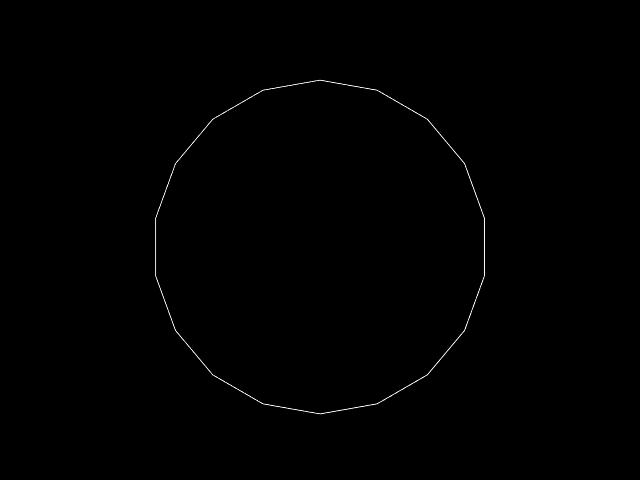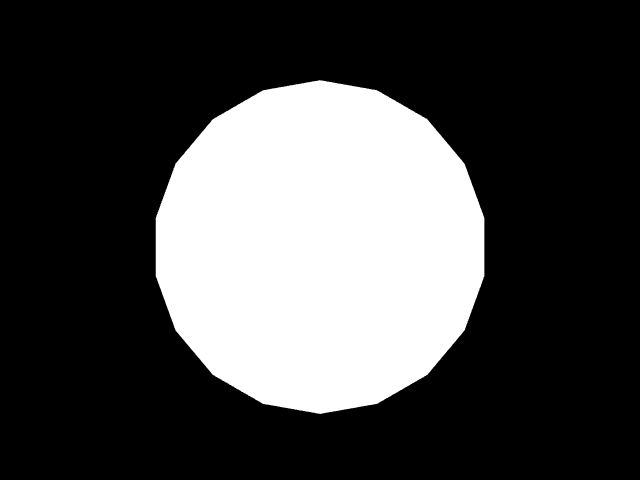# Some other features

(back to contents)

## Polygons

"The Algorithmic Beauty of Plants" book describes a way to represent procedural surface models by making turtle draw polygons.```(with-graphics (iteration-count 18) (set-axiom (turn(-80) A)) (add-rule (A -> forward(100) turn(20) A))) ```

This example draws the familiar outline of a regular polygon.```(with-graphics (iteration-count 18) (set-axiom ({ turn(-80) A })) (add-rule (A -> edge(100) turn(20) A))) ```

This example draws the polygon itself. Here are command explanations:

 `{` - start polygon shape `}` - end polygon shape `dot` - draw polygon point `edge` - draw polygon edge
Each polygon must start with `{` and must end with `}`, `{` and `}` can be nested. In between `{` and `}` there must be at least three `dot` or `edge` commands. `(edge(x))` is essentially the same as `(jump(x) dot)`. The reason why `dot` is separated is that normal vector for a polygon vertex is assumed to be turtle's current local Y axis. Perhaps it is convenient to change turtle orientation before issuing `dot`, for example `(jump(100) turn(10) dot turn(10) jump(100) ...)`.

## Ripping branches

There are times when it is necessary to destroy whole branches — erase lots of different symbols at once. For that there is a `rip` command which erases everything up until matching closing square bracket. Consider example:

```(with-debug
(iteration-count 2)
(set-axiom (B C C [ C X C B [ B B C ] C B ] C C B))
```
which rewrites in the following manner:
 axiom: `(B C C [ C X C B [ B B C ] C B ] C C B)` p1: `(B C C [ C Y C B [ B B C ] C B ] C C B)` p2: `(B C C [ C ] C C B)`
In L-systems similar to the one in chapter four where weights of branches are propagated, `rip` command could be used to snap branches that get too heavy.

## Meta rules

LISP makes it possible to pass down rules as arguments:

```(with-graphics
(iteration-count 19)
(set-axiom (turn(-80) A))
(add-rule (A -> meta-rule(#'F 100) meta-rule(#'turn 20) A))
(add-rule (meta-rule(rule parameter) -> funcall(rule parameter))))
```
This code produces same circle outline that is shown above.
Try out another LISPy example:
```(add-rule ((delay(rule args time) ? (> time 0) -> delay(rule args (1- time)))
(delay(rule args time) ? (= time 0) -> apply(rule args))))

```

(back to contents)